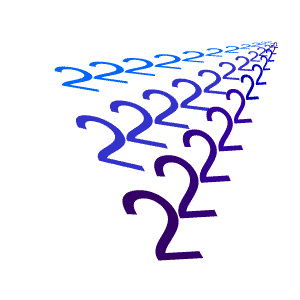#### You may also likeMake a set of numbers that use all the digits from 1 to 9, once and once only. Add them up. The result is divisible by 9. Add each of the digits in the new number. What is their sum? Now try some other possibilities for yourself!### What an Odd Fact(or)

Can you show that 1^99 + 2^99 + 3^99 + 4^99 + 5^99 is divisible by 5?### Counting Factors

Is there an efficient way to work out how many factors a large number has?

# Remainder

##### Age 11 to 14 Challenge Level:

What is the remainder of $2^{2002}\div 7?$What happens with different powers of $2$?

Try to explain the mathematics behind what you discover.